Function Repository Resource:

# ObjectDetectionAnchorBoxes

Create an array of candidate bounding boxes for object detection (anchors)

Contributed by: Davit Baghdasaryan
 ResourceFunction["ObjectDetectionAnchorBoxes"][{w,h},{s1,s2,…},{r1,r2,…}] computes the array of anchor boxes with combinations of sizes si and aspect ratios ri for each cell in a grid with size {w,h}.

## Details and Options

Ratios ri and sizes si must be real values from 0 to 1.
The number of anchors centered on each cell of a grid is generated with width and height {si*Sqrt[rj],si/Sqrt[rj]} with different combinations of si and rj.
Anchor coordinates values typically are from 0 to 1.
The following options are supported:
 Method Full the method to generate anchors per pixel "ClippingQ" False whether to clip out-of-boundary anchors "Steps" Automatic gird steps in vertical and horizontal directions "Offsets" {0.5,0.5} anchor offsets from the bottom left corner of a grid cell "CoordinateSystem" “ReversedGraphicsCoordinates" coordinate system of the anchors "BoundingBoxFormat" "Corner" anchor format: "Corner", "Center" or "Rectangle"
"CoordinateSystem" can be "GraphicsCoordinates" with origin in the bottom left corner or "ReversedGraphicsCoordinates" with origin in the top left corner.
Cells are sorted from left to right, from top to bottom independent of the "CoordinateSystem" value.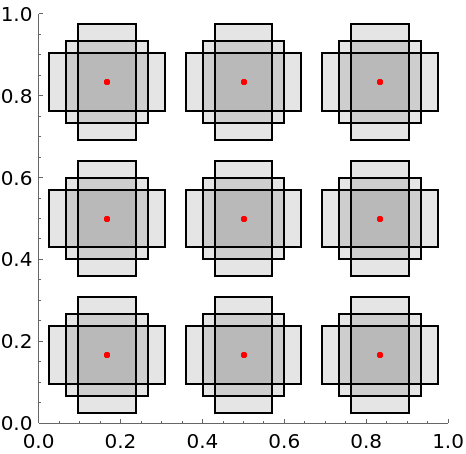If Method option is “SSD", size and ratio combinations are {si*Sqrt[r1],si/Sqrt[r1]}, and {s1*Sqrt[rj],s1/Sqrt[rj]}, with i from 1 to n, j from 2 to m, with total number n+m-1, and where n and m are the length of the scale and ratios lists. If Method is Full, all combinations of sizes and ratios are used with a total number n*m per cell.
For "BoundingBoxFormat""Corner", the result is a list of the form {xmin,ymin,xmax,ymax} while for "BoundingBoxFormat""Center", the result is in the form {xcenter,ycenter,width,height}.
Setting “BoundingBoxFormat to "Rectangle"returns list of Rectangle objects.

## Examples

### Basic Examples (3)

Generate anchor box coordinates:

 In:=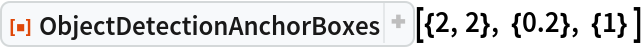Out=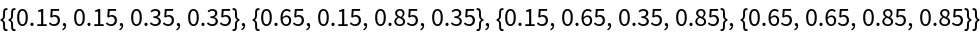Generate the anchor boxes in Rectangle format and then show them using Graphics function:

 In:=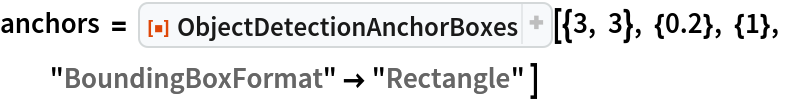Out=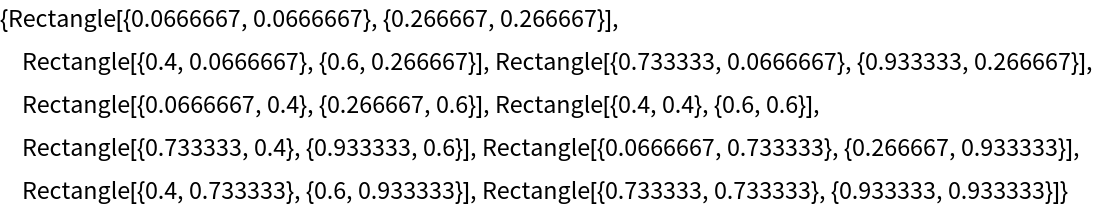In:=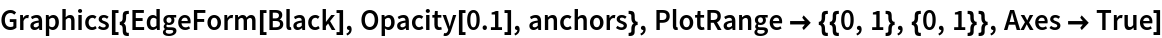Out=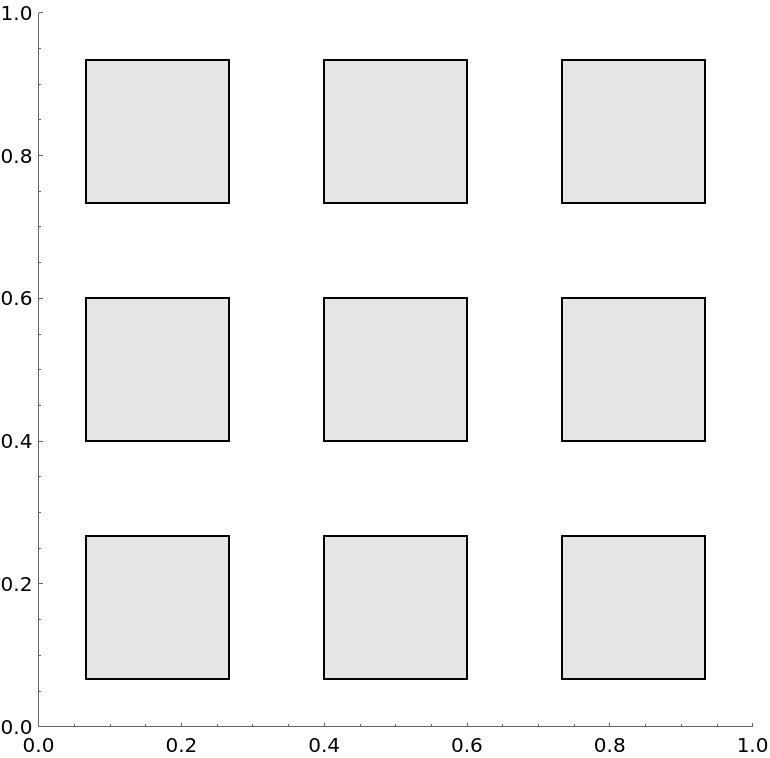Generate the anchor boxes for 3 by 3 grid:

 In:=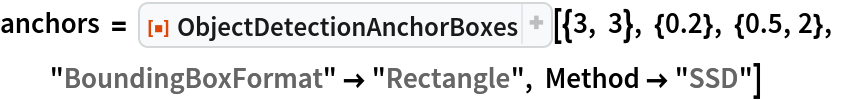Out=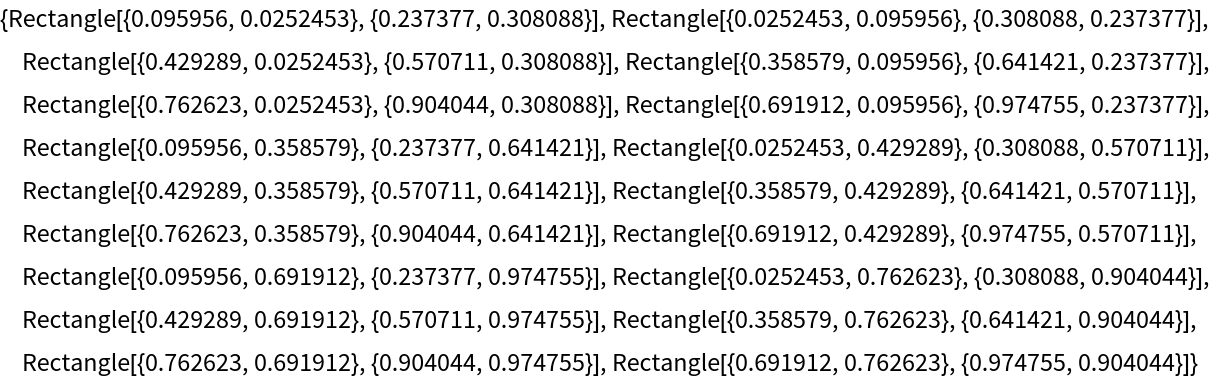In:=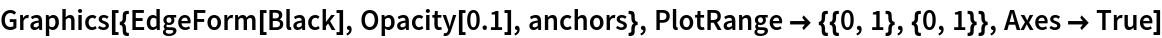Out=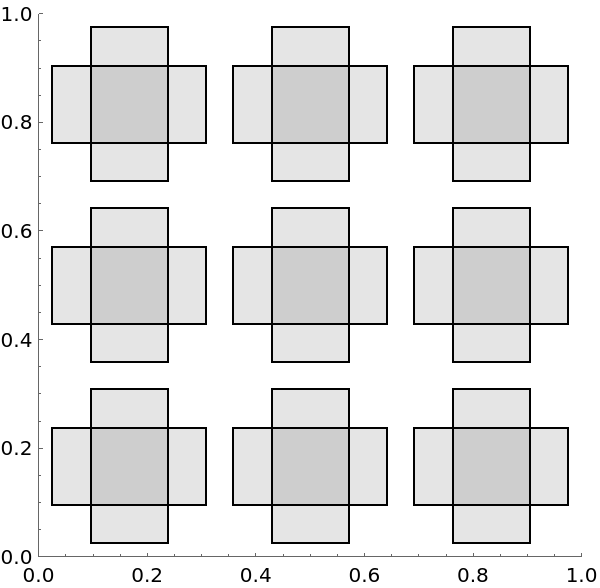### Scope (5)

Generate and visualize anchors with one size and one ratio:

 In:=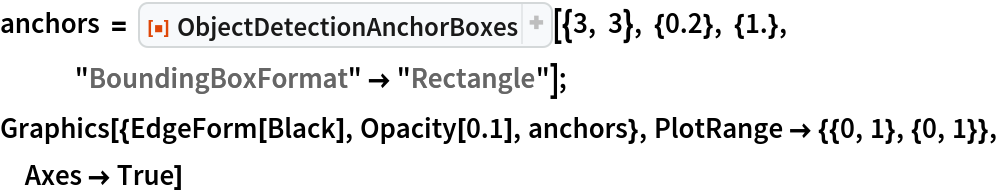Out=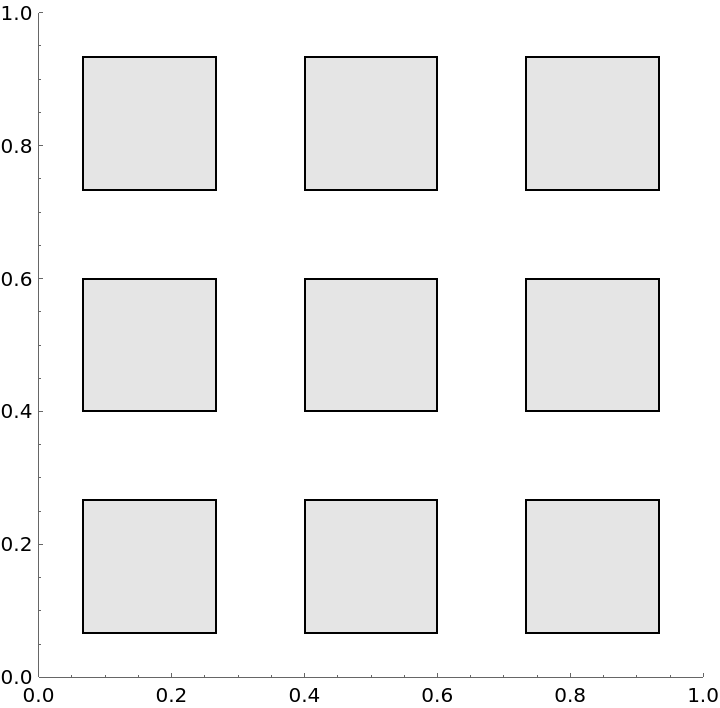Generate anchors with two sizes and one ratio:

 In:=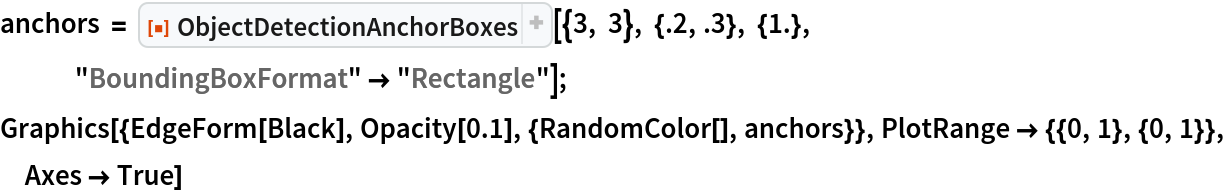Out=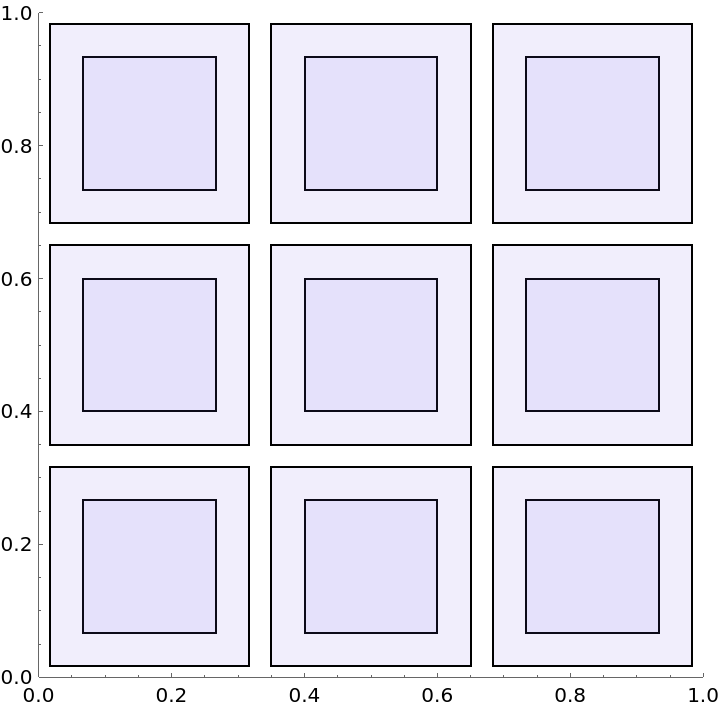Generate anchors with two ratios and one size:

 In:=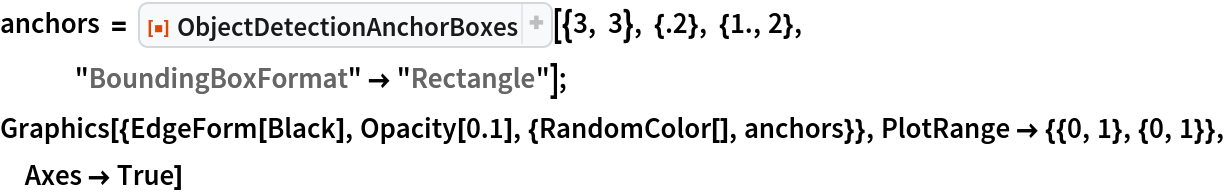Out=Note that generating the Anchors with two aspect ratios and two sizes leads to 3 anchors per pixel because of the default Method-> "SSD" (see Options section):

 In:=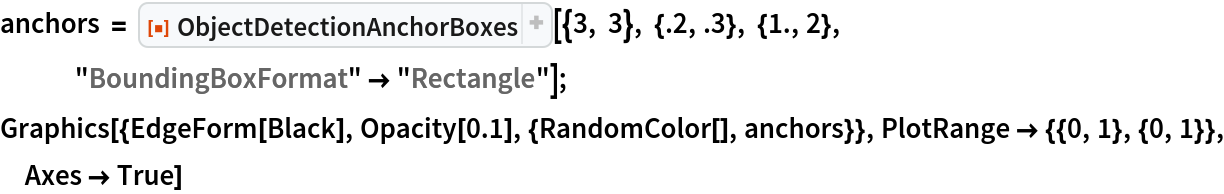Out=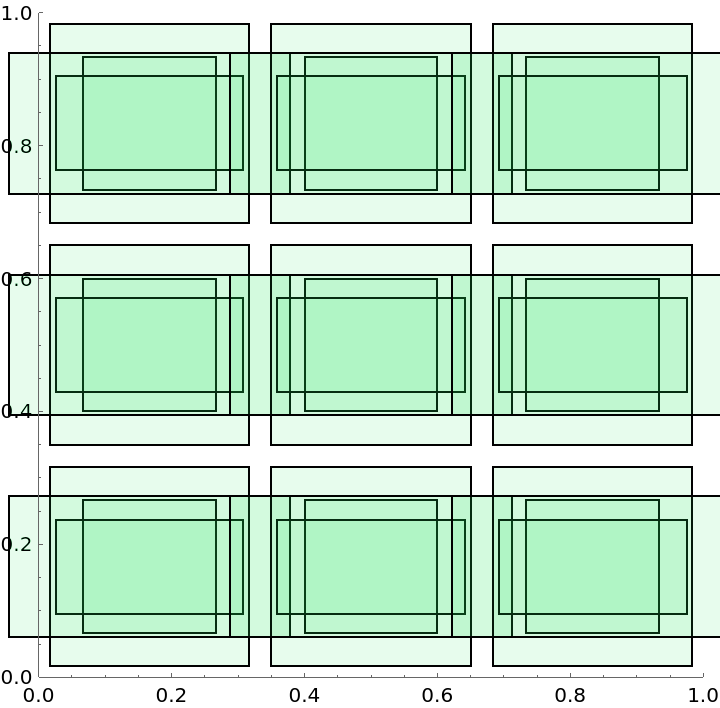In:=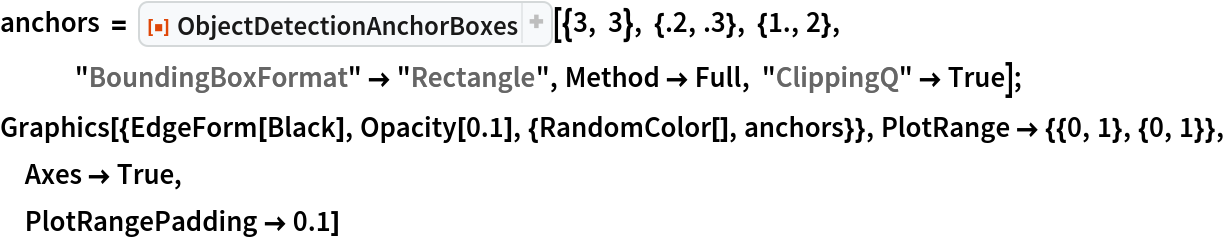Out=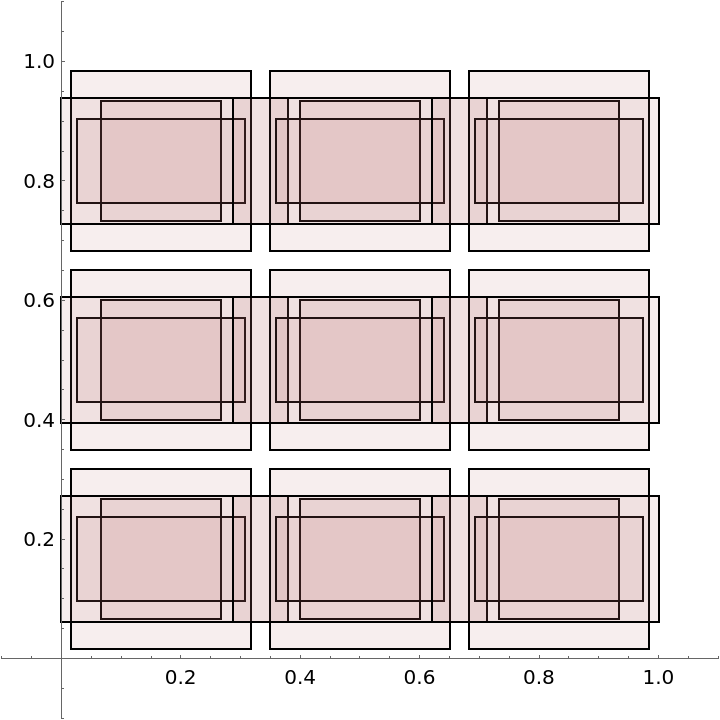### Options (7)

#### Method (2)

With the default method all combinations of the sizes and aspect ratios are used:

 In:=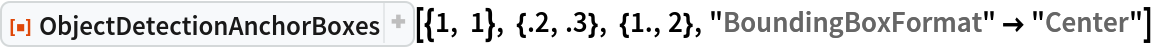Out=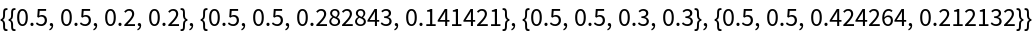With the "SSD" Method option only the first size and first ratio are combined with the others:

 In:=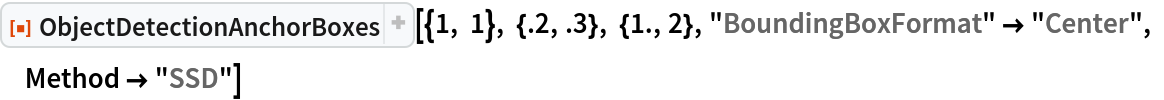Out=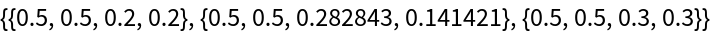#### ClippingQ (2)

If the scale of the bounding boxes is large enough then it can be out of the image boundary:

 In:=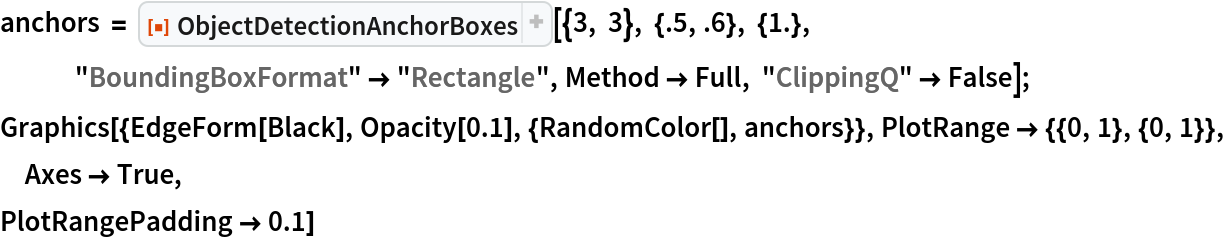Out=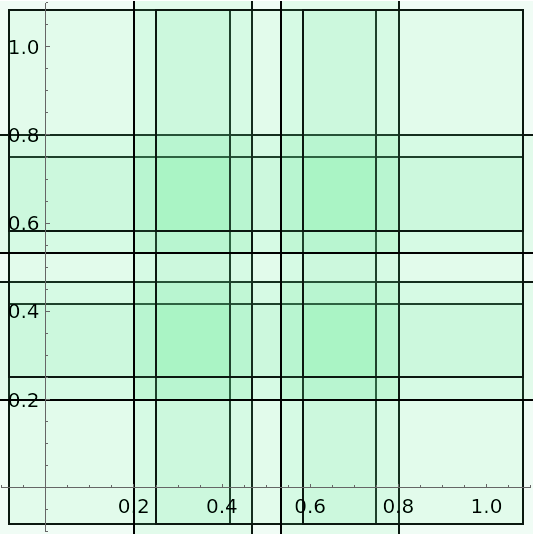The above can be fixed by specifying the "ClippingQ" option to True to clip the bounding boxes:

 In:=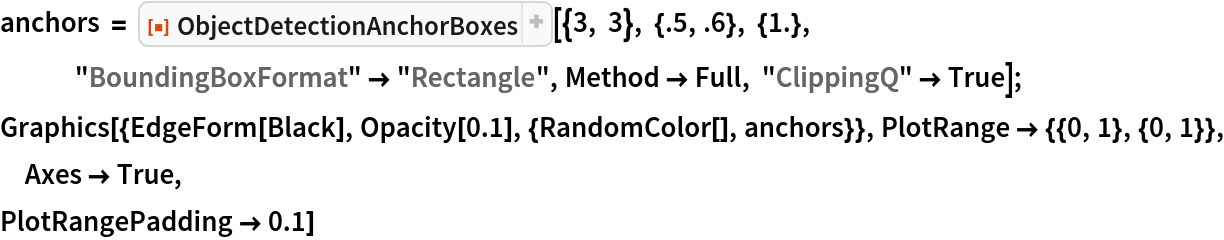Out=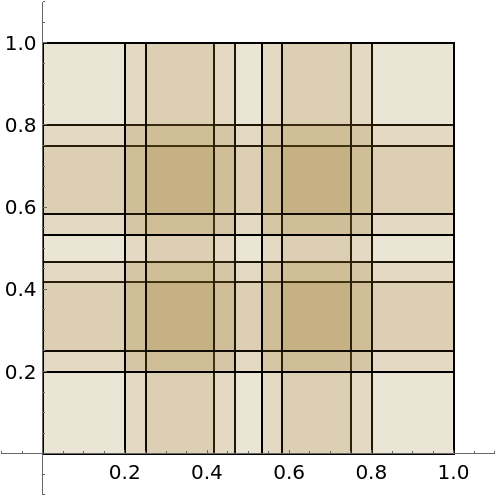#### BoundingBoxFormat (3)

By default bounding boxes are returned as {xmin,ymin,xmax,ymax}:

 In:=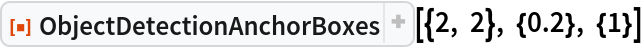Out=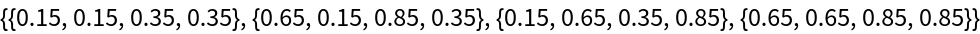Use "BoundingBoxFormat""Center" to create anchors in the format {xcenter,ycenter,w,h}:

 In:=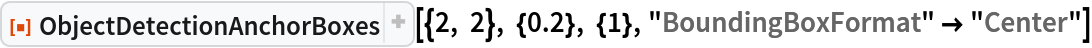Out=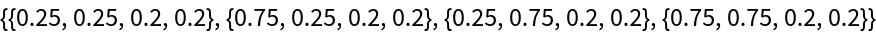Use "BoundingBoxFormat""Rectangle" to create anchor in the format of Rectangle[{xmin,ymin},{xmax,ymax}]:

 In:=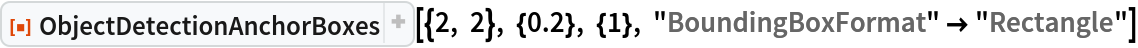Out=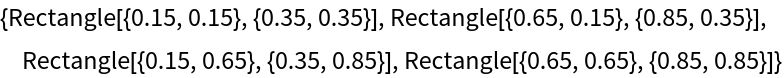### Neat Examples (2)

Retrieve a large image:

 In:=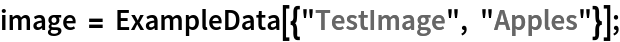In:=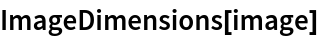Out=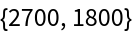Create image patches using ObjectDetectionAnchorBoxes:

 In:=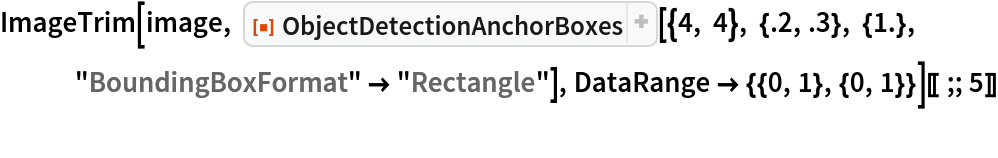Out=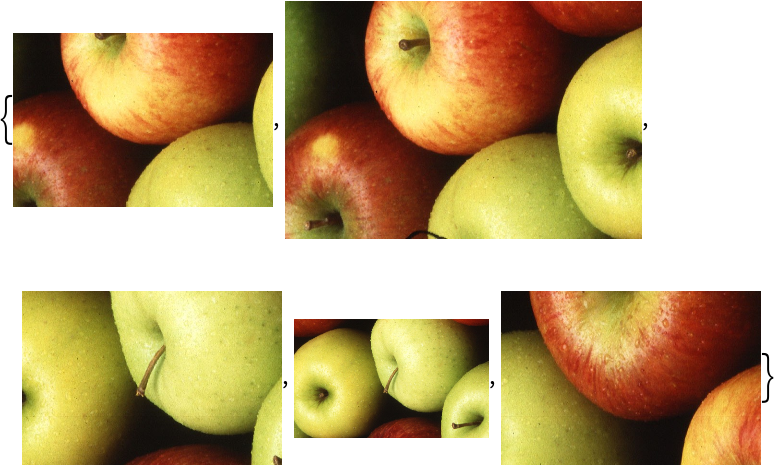## Version History

• 1.0.0 – 22 March 2022